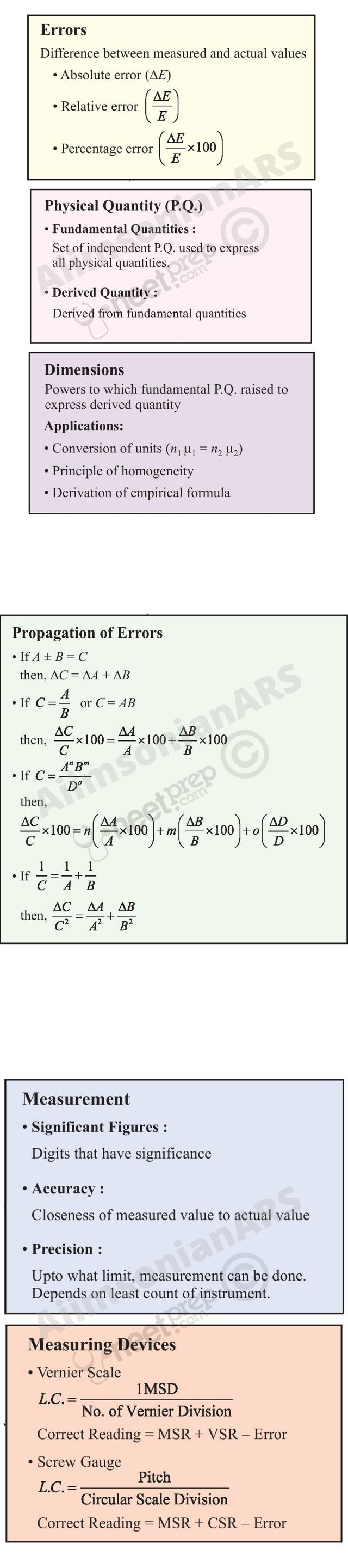# Units and Measurement

2.1 Introduction

2.2 The international system of units

2.3 Measurement of length

2.4 Measurement of mass

2.5 Measurement of time

2.6 Accuracy, precision of instruments and errors in measurement

2.7 Significant figures

2.8 Dimensions of physical quantities

2.9 Dimensional formulae and dimensional equations

2.10 Dimensional analysis and its applications

Summary

Exercises

## 2.1 Introduction

Measurement of any physical quantity involves comparison with a certain basic, arbitrarily chosen, internationally accepted reference standard called unit. The result of a measurement of a physical quantity is expressed by a number (or numerical measure) accompanied by a unit. Although the number of physical quantities appears to be very large, we need only a limited number of units for expressing all the physical quantities, since they are inter-related with one another. The units for the fundamental or base quantities are called fundamental or base units. The units of all other physical quantities can be expressed as combinations of the base units. Such units obtained for the derived quantities are called derived units. A complete set of these units, both the base units and derived units, is known as the system of units.

## 2.2 The International System of Units

In earlier time scientists of different countries were using different systems of units for measurement. Three such systems, the CGS, the FPS (or British) system and the MKS system were in use extensively till recently.

The base units for length, mass and time in these systems were as follows :

In CGS system they were centimetre, gram and second respectively.

In FPS system they were foot, pound and second respectively.

In MKS system they were metre, kilogram and second respectively.

The system of units which is at present internationally accepted for measurement is the Système Internationale d’ Unites (French for International System of Units), abbreviated as SI. The SI, with standard scheme of symbols, units and abbreviations, was developed and recommended by General Conference on Weights and Measures in 1971 for international usage in scientific, technical, industrial and commercial work. Because SI units used decimal system, conversions within the system are quite simple and convenient. We shall follow the SI units in this book.

In SI, there are seven base units as given in Table 2.1. Besides the seven base units, there are two more units that are defined for (a) plane angle dθ as the ratio of length of arc ds to the radius r and (b) solid angle d as the ratio of the intercepted area dA of the spherical surface, described about the apex O as the centre, to the square of its radius r, as shown in Fig. 2.1(a) and (b) respectively. The unit for plane angle is radian with the symbol rad and the unit for the solid angle is steradian with the symbol sr. Both these are dimensionless quantities.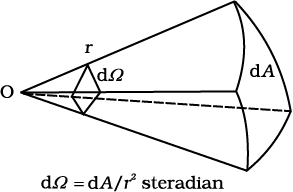(a)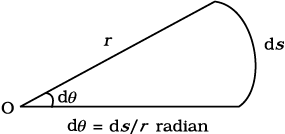(b)
Fig. 2.1 Description of (a) plane angle dθ and (b) solid angle dΩ
##### Table 2.1SI Base Quantities and Units*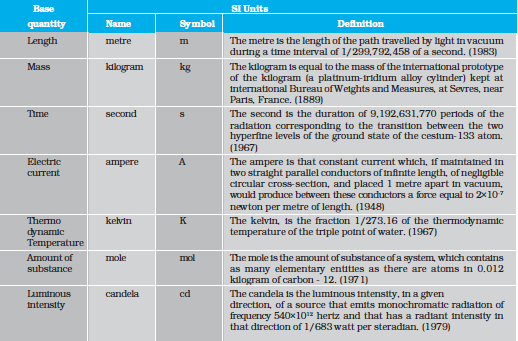* The values mentioned here need not be remembered or asked in a test. They are given here only to indicate the extent of accuracy to which they are measured. With progress in technology, the measuring techniques get improved leading to measurements with greater precision. The definitions of base units are revised to keep up with this progress.

Note that when mole is used, the elementary entities must be specified. These entities may be atoms, molecules, ions, electrons, other particles or specified groups of such particles.

##### Table 2.2 Some units retained for general use (Though outside SI)We employ units for some physical quantities that can be derived from the seven base units (Appendix A 6). Some derived units in terms of the SI base units are given in (Appendix A 6.1). Some SI derived units are given special names (Appendix A 6.2 ) and some derived SI units make use of these units with special names and the seven base units (Appendix A 6.3). These are given in Appendix A 6.2 and A 6.3 for your ready reference. Other units retained for general use are given in Table 2.2.

Common SI prefixes and symbols for multiples and sub-multiples are given in Appendix A2. General guidelines for using symbols for physical quantities, chemical elements and nuclides are given in Appendix A7 and those for SI units and some other units are given in Appendix A8 for your guidance and ready reference.

## 2.3 Measurement of Length

You are already familiar with some direct methods for the measurement of length. For example, a metre scale is used for lengths from 10–3 m to 102 m. A vernier callipers is used for lengths to an accuracy of 10–4 m. A screw gauge and a spherometer can be used to measure lengths as less as to 10–5 m. To measure lengths beyond these ranges, we make use of some special indirect methods.

### 2.3.1 Measurement of Large Distances

Large distances such as the distance of a planet or a star from the earth cannot be measured directly with a metre scale. An important method in such cases is the parallax method.

When you hold a pencil in front of you against some specific point on the background (a wall) and look at the pencil first through your left eye A (closing the right eye) and then look at the pencil through your right eye B (closing the left eye), you would notice that the position of the pencil seems to change with respect to the point on the wall. This is called parallax. The distance between the two points of observation is called the basis. In this example, the basis is the distance between the eyes.

To measure the distance D of a faraway planet S by the parallax method, we observe it from two different positions (observatories) A and B on the Earth, separated by distance AB = b at the same time as shown in Fig. 2.2. We measure the angle between the two directions along which the planet is viewed at these two points. The ASB in Fig. 2.2 represented by symbol θ is called the parallax angle or parallactic angle.

As the planet is very far away,b/D<<1, and therefore, θ is very small. Then we approximately take AB as an arc of length b of a circle with centre at S and the distance D as the radius AS = BS so that AB = b = D θ where θ is in radians.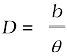(2.1)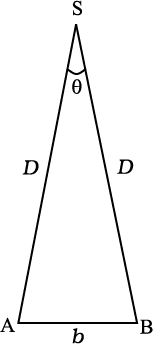Fig. 2.2 Parallax method.

Having determined D, we can employ a similar method to determine the size or angular diameter of the planet. If d is the diameter of the planet and α the angular size of the planet (the angle subtended by d at the earth), we have

α = d/D                                                                (2.2)

The angle α can be measured from the same location on the earth. It is the angle between the two directions when two diametrically opposite points of the planet are viewed through the telescope. Since D is known, the diameter d of the planet can be determined using Eq. (2.2).

Example 2.1 Calculate the angle of (a) 10 (degree) (b) 1 (minute of arc or arcmin) and (c) 1(second of arc or arc second) in radians. Use 3600=2π rad, 10=60 and  1 = 60

(a) We have 3600 = 2π rad

(b) 10 = 60= 1.745×10–2 rad

Example 2.2 A man wishes to estimate the distance of a nearby tower from him. He stands at a point A in front of the tower C and spots a very distant object O in line with AC. He then walks perpendicular to AC up to B, a distance of 100 m, and looks at O and C again. Since O is very distant, the direction BO is practically the same as AO; but he finds the line of sight of C shifted from the original line of sight by an angle θ = 400 (θ is known as ‘parallax’) estimate the distance of the tower C from his original position A.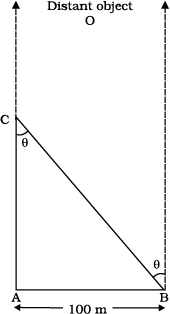Fig. 2.3

We have, parallax angle θ = 400

From Fig. 2.3, AB = AC tan θ

AC = AB/tanθ = 100 m/tan 400

= 100 m/0.8391 = 119 m

Example 2.3 The moon is observed from two diametrically opposite points A and B on Earth. The angle θ subtended at the moon by the two directions of observation is 1o 54. Given the diameter of the Earth to be about 1.276 × 107 m, compute the distance of the moon from the Earth.

We have θ = 1° 54 = 114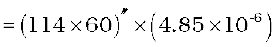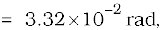since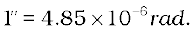Also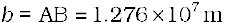Hence from Eq. (2.1), we have the earth-moon distance,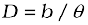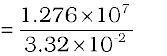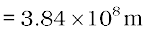Example 2.4 The Sun’s angular diameter is measured to be 1920′′. The distance D of the Sun from the Earth is 1.496 × 1011 m. What is the diameter of the Sun ?

Sun’s angular diameter α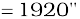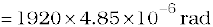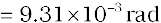Sun’s diameter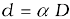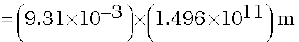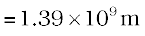### 2.3.2Estimation of Very Small Distances: Size of a Molecule

To measure a very small size, like that of a molecule (10–8 m to 10–10 m), we have to adopt special methods. We cannot use a screw gauge or similar instruments. Even a microscope has certain limitations. An optical microscope uses visible light to ‘look’ at the system under investigation. As light has wave like features, the resolution to which an optical microscope can be used is the wavelength of light (A detailed explanation can be found in the Class XII Physics textbook). For visible light the range of wavelengths is from about 4000 Å to 7000 Å
(1 angstrom = 1 Å = 10
-10 m). Hence an optical microscope cannot resolve particles with sizes smaller than this. Instead of visible light, we can use an electron beam. Electron beams can be focussed by properly designed electric and magnetic fields. The resolution of such an electron microscope is limited finally by the fact that electrons can also behave as waves ! (You will learn more about this in class XII). The wavelength of an electron can be as small as a fraction of an angstrom. Such electron microscopes with a resolution of 0.6 Å have been built. They can almost resolve atoms and molecules in a material. In recent times, tunnelling microscopy has been developed in which again the limit of resolution is better than an angstrom. It is possible to estimate the sizes of molecules.

A simple method for estimating the molecular size of oleic acid is given below. Oleic acid is a soapy liquid with large molecular size of the order of 10–9 m.

The idea is to first form mono-molecular layer of oleic acid on water surface.

We dissolve 1 cm3 of oleic acid in alcohol to make a solution of 20 cm3. Then we take 1 cm3 of this solution and dilute it to 20 cm3, using alcohol. So, the concentration of the solution is equal to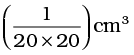of oleic acid/cm3 of solution. Next we lightly sprinkle some lycopodium powder on the surface of water in a large trough and we put one drop of this solution in the water. The oleic acid drop spreads into a thin, large and roughly circular film of molecular thickness on water surface. Then, we quickly measure the diameter of the thin film to get its area A. Suppose we have dropped n drops in the water. Initially, we determine the approximate volume of each drop (V cm3).

Volume of n drops of solution

= nV cm3

Amount of oleic acid in this solution

=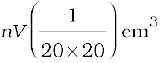This solution of oleic acid spreads very fast on the surface of water and forms a very thin layer of thickness t. If this spreads to form a film of area A cm2, then the thickness of the film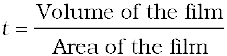or,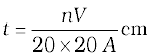(2.3)

If we assume that the film has mono-molecular thickness, then this becomes the size or diameter of a molecule of oleic acid. The value of this thickness comes out to be of the order of 10–9 m.

Example 2.5 If the size of a nucleus (in the range of 10–15 to 10–14 m) is scaled up to the tip of a sharp pin, what roughly is the size of an atom ? Assume tip of the pin to be in the range 10–5m to 10–4m.

Answer  The size of a nucleus is in the range of 10–15 m and 10–14 m. The tip of a sharp pin is taken to be in the range of 10–5 m and 10–4 m. Thus we are scaling up by a factor of 1010. An atom roughly of size 10–10 m will be scaled up to a size of 1 m. Thus a nucleus in an atom is as small in size as the tip of a sharp pin placed at the centre of a sphere of radius about a metre long. t

### 2.3.3 Range of Lengths

The sizes of the objects we come across in the universe vary over a very wide range. These may vary from the size of the order of 10–14 m of the tiny nucleus of an atom to the size of the order of 1026 m of the extent of the observable universe. Table 2.3 gives the range and order of lengths and sizes of some of these objects.

We also use certain special length units for short and large lengths. These are

1 fermi = 1 f = 10–15 m

1 angstrom = 1 Å = 10–10 m

1 astronomical unit = 1 AU (average distance of the Sun from the Earth)

= 1.496 × 1011 m

1 light year = 1 ly = 9.46 × 1015 m (distance that light travels with velocity of 3 × 108 m s–1 in 1 year)

1 parsec = 3.08 × 1016 m (Parsec is the distance at which average radius of earth’s orbit subtends an angle of 1 arc second)

## 2.4 Measurement of Mass

Mass is a basic property of matter. It does not depend on the temperature, pressure or location of the object in space. The SI unit of mass is kilogram (kg). The prototypes of the International standard kilogram supplied by the International Bureau of Weights and Measures (BIPM) are available in many other laboratories of different countries. In India, this is available at the National Physical Laboratory (NPL), New Delhi.

While dealing with atoms and molecules, the kilogram is an inconvenient unit. In this case, there is an important standard unit of mass, called the unified atomic mass unit (u), which has been established for expressing the mass of atoms as

1 unified atomic mass unit = 1u

= (1/12) of the mass of an atom of carbon-12 isotope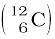including the mass of electrons

= 1.66 × 10–27 kg

Mass of commonly available objects can be determined by a common balance like the one used in a grocery shop. Large masses in the universe like planets, stars, etc., based on Newton’s law of gravitation can be measured by using gravitational method (See Chapter 8). For measurement of small masses of atomic/sub-atomic particles etc., we make use of mass spectrograph in which radius of the trajectory is proportional to the mass of a charged particle moving in uniform electric and magnetic field.

### 2.4.1 Range of Masses

The masses of the objects, we come across in the universe, vary over a very wide range. These may vary from tiny mass of the order of 10-30 kg of an electron to the huge mass of about 1055 kg of the known universe. Table 2.4 gives the range and order of the typical masses of various objects.

##### Table 2.3 Range and order of lengths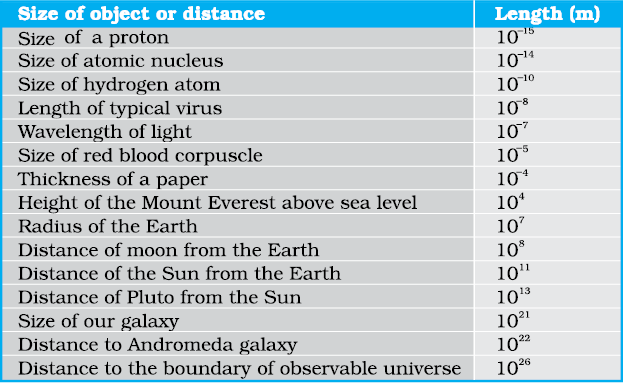## 2.5 Measurement of time

To measure any time interval we need a clock. We now use an atomic standard of time, which is based on the periodic vibrations produced in a cesium atom. This is the basis of the cesium clock, sometimes called atomic clock, used in the national standards. Such standards are available in many laboratories. In the cesium atomic clock, the second is taken as the time needed for 9,192,631,770 vibrations of the radiation corresponding to the transition between the two hyperfine levels of the ground state of cesium-133 atom. The vibrations of the cesium atom regulate the rate of this cesium atomic clock just as the vibrations of a balance wheel regulate an ordinary wristwatch or the vibrations of a small quartz crystal regulate a quartz wristwatch.

The cesium atomic clocks are very accurate. In principle they provide portable standard. The national standard of time interval ‘second’ as well as the frequency is maintained through four cesium atomic clocks. A cesium atomic clock is used at the National Physical Laboratory (NPL), New Delhi to maintain the Indian standard of time.

In our country, the NPL has the responsibility of maintenance and improvement of physical standards, including that of time, frequency, etc. Note that the Indian Standard Time (IST) is linked to this set of atomic clocks. The efficient cesium atomic clocks are so accurate that they impart the uncertainty in time realisation as ± 1 × 10–13, i.e. 1 part in 1013. This implies that the uncertainty gained over time by such a device is less than 1 part in 1013; they lose or gain no more than 3 µs in one year. In view of the tremendous accuracy in time measurement, the SI unit of length has been expressed in terms the path length light travels in certain interval of time (1/299, 792, 458 of a second) (Table 2.1).

The time interval of events that we come across in the universe vary over a very wide range. Table 2.5 gives the range and order of some typical time intervals.

You may notice that there is an interesting coincidence between the numbers appearing in Tables 2.3 and 2.5. Note that the ratio of the longest and shortest lengths of objects in our universe is about 1041. Interestingly enough, the ratio of the longest and shortest time intervals associated with the events and objects in our universe is also about 1041. This number, 1041 comes up again in Table 2.4, which lists typical masses of objects. The ratio of the largest and smallest masses of the objects in our universe is about (1041)2. Is this a curious coincidence between these large numbers purely accidental ?

## 2.6 Accuracy, precision of instruments and errors in measurement

Measurement is the foundation of all experimental science and technology. The result of every measurement by any measuring instrument contains some uncertainty. This uncertainty is called error. Every calculated quantity which is based on measured values, also has an error. We shall distinguish between two terms: accuracy and precision. The accuracy of a measurement is a measure of how close the measured value is to the true value of the quantity. Precision tells us to what resolution or limit the quantity is measured.

The accuracy in measurement may depend on several factors, including the limit or the resolution of the measuring instrument. For example, suppose the true value of a certain length is near 3.678 cm. In one experiment, using a measuring instrument of resolution 0.1 cm, the measured value is found to be 3.5 cm, while in another experiment using a measuring device of greater resolution, say 0.01 cm, the length is determined to be 3.38 cm. The first measurement has more accuracy (because it is closer to the true value) but less precision (its resolution is only 0.1 cm), while the second measurement is less accurate but more precise. Thus every measurement is approximate due to errors in measurement. In general, the errors in measurement can be broadly classified as (a) systematic errors and (b) random errors.

##### Table 2.5 Range and order of time intervals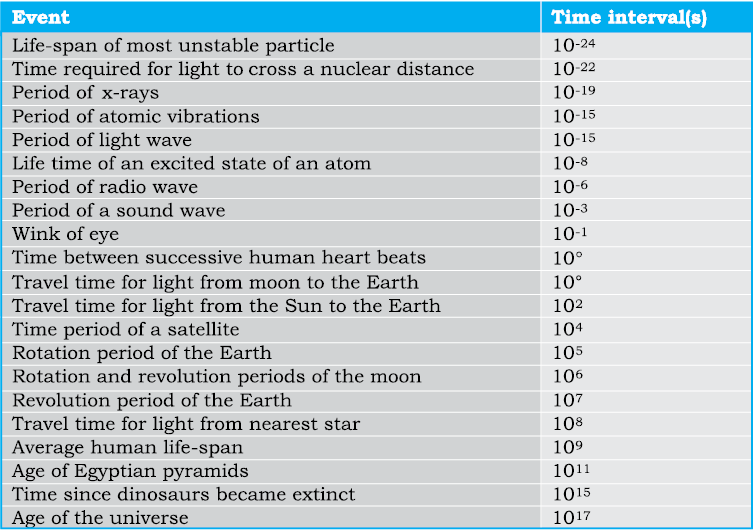Systematic errors

The systematic errors are those errors that tend to be in one direction, either positive or negative. Some of the sources of systematic errors are :

(a) Instrumental errors that arise from the errors due to imperfect design or calibration of the measuring instrument, zero error in the instrument, etc. For example, the temperature graduations of a thermometer may be inadequately calibrated (it may read 104 °C at the boiling point of water at STP whereas it should read 100 °C); in a vernier callipers the zero mark of vernier scale may not coincide with the zero mark of the main scale, or simply an ordinary metre scale may be worn off at one end.

(b) Imperfection in experimental technique or procedure To determine the temperature of a human body, a thermometer placed under the armpit will always give a temperature lower than the actual value of the body temperature. Other external conditions (such as changes in temperature, humidity, wind velocity, etc.) during the experiment may systematically affect the measurement.

(c) Personal errors that arise due to an individual’s bias, lack of proper setting of the apparatus or individual’s carelessness in taking observations without observing proper precautions, etc. For example, if you, by habit, always hold your head a bit too far to the right while reading the position of a needle on the scale, you will introduce an error due to parallax.

Systematic errors can be minimised by improving experimental techniques, selecting better instruments and removing personal bias as far as possible. For a given set-up, these errors may be estimated to a certain extent and the necessary corrections may be applied to the readings.

Random errors

The random errors are those errors, which occur irregularly and hence are random with respect to sign and size. These can arise due to random and unpredictable fluctuations in experimental conditions (e.g. unpredictable fluctuations in temperature, voltage supply, mechanical vibrations of experimental set-ups, etc), personal (unbiased) errors by the observer taking readings, etc. For example, when the same person repeats the same observation, it is very likely that he may get different readings everytime.

Least count error

The smallest value that can be measured by the measuring instrument is called its least count. All the readings or measured values are good only up to this value.

The least count error is the error associated with the resolution of the instrument. For example, a vernier callipers has the least count as 0.01cm; a spherometer may have a least count of 0.001 cm. Least count error belongs to the category of random errors but within a limited size; it occurs with both systematic and random errors. If we use a metre scale for measurement of length, it may have graduations at 1 mm division scale spacing or interval.

Using instruments of higher precision, improving experimental techniques, etc., we can reduce the least count error. Repeating the observations several times and taking the arithmetic mean of all the observations, the mean value would be very close to the true value of the measured quantity.

### 2.6.1 Absolute Error, Relative Error and Percentage Error

(a)

Suppose the values obtained in several measurements are a1, a2, a3...., an. The arithmetic mean of these values is taken as the best possible value of the quantity under the given conditions of measurement as :

amean = (a1+a2+a3+...+an ) / n                                                                                         (2.4)

or,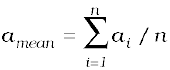(2.5)

This is because, as explained earlier, it is reasonable to suppose that individual measurements are as likely to overestimate as to underestimate the true value of the quantity.

The magnitude of the difference between the individual measurement and the true value of the quantity is called the absolute error of the measurement. This is denoted by |∆a |. In absence of any other method of knowing true value, we considered arithmatic mean as the true value. Then the errors in the individual measurement values from the true value, are

a1 = a1amean,

a2 = a2amean,

.... .... ....

.... .... ....

a n = anamean

The a calculated above may be positive in certain cases and negative in some other cases. But absolute error |a| will always be positive.

(b) The arithmetic mean of all the absolute errors is taken as the final or mean absolute error of the value of the physical quantity a. It is represented by amean.

Thus,

amean = (|a1|+|a2 |+|a3|+...+ |an|)/n                                                                      (2.6)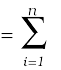|ai|/n                                                                                                               (2.7)

If we do a single measurement, the value we get may be in the range amean ± amean

i.e. a = amean ± amean

or,

ameanamean a amean + amean                                                                       (2.8)

This implies that any measurement of the physical quantity a is likely to lie between (amean+amean) and (amean− ∆amean).

(c) Instead of the absolute error, we often use the relative error or the percentage error (δa). The relative error is the ratio of the mean absolute error amean to the mean value amean of the quantity measured.

Relative error = amean/amean                                                                                        (2.9)

When the relative error is expressed in per cent, it is called the percentage error (δa).

Thus, Percentage error

δa = (amean/amean) × 100%                                                                                          (2.10)

Let us now consider an example.

Example 2.6 Two clocks are being tested against a standard clock located in a national laboratory. At 12:00:00 noon by the standard clock, the readings of the two clocks are :

Clock 1                             Clock 2

Monday                                  12:00:05                           10:15:06

Tuesday                                 12:01:15                           10:14:59

Wednesday                            11:59:08                           10:15:18

Thursday                                12:01:50                           10:15:07

Friday                                     11:59:15                           10:14:53

Saturday                                 12:01:30                           10:15:24

Sunday                                   12:01:19                           10:15:11

If you are doing an experiment that requires precision time interval measurements, which of the two clocks will you prefer ?

The range of variation over the seven days of observations is 162 s for clock 1, and 31 s for clock 2. The average reading of clock 1 is much closer to the standard time than the average reading of clock 2. The important point is that a clock’s zero error is not as significant for precision work as its variation, because a ‘zero-error’ can always be easily corrected. Hence clock 2 is to be preferred to clock 1.

Example 2.7 We measure the period of oscillation of a simple pendulum. In successive measurements, the readings turn out to be 2.63 s, 2.56 s, 2.42 s, 2.71s and 2.80 s. Calculate the absolute errors, relative error or percentage error.

Answer The mean period of oscillation of the pendulum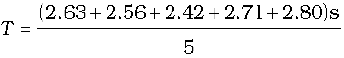=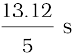= 2.624 s

= 2.62 s

As the periods are measured to a resolution of 0.01 s, all times are to the second decimal; it is proper to put this mean period also to the second decimal.

The errors in the measurements are

2.63 s – 2.62 s = 0.01 s

2.56 s – 2.62 s = – 0.06 s

2.42 s – 2.62 s = – 0.20 s

2.71 s – 2.62 s = 0.09 s

2.80 s – 2.62 s = 0.18 s

Note that the errors have the same units as the quantity to be measured.

The arithmetic mean of all the absolute errors (for arithmetic mean, we take only the magnitudes) is

∆Τmean = [(0.01+ 0.06+0.20+0.09+0.18)s]/5

= 0.54 s/5

= 0.11 s

That means, the period of oscillation of the simple pendulum is (2.62 ± 0.11) s i.e. it lies between (2.62 + 0.11) s and (2.62 – 0.11) s or between 2.73 s and 2.51 s. As the arithmetic mean of all the absolute errors is 0.11 s, there is already an error in the tenth of a second. Hence there is no point in giving the period to a hundredth. A more correct way will be to write

T = 2.6 ± 0.1 s

Note that the last numeral 6 is unreliable, since it may be anything between 5 and 7. We indicate this by saying that the measurement has two significant figures. In this case, the two significant figures are 2, which is reliable and 6, which has an error associated with it. You will learn more about the significant figures in section 2.7.

For this example, the relative error or the percentage error is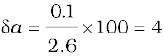### 2.6.2 Combination of Errors

If we do an experiment involving several measurements, we must know how the errors in all the measurements combine. For example, mass density is obtained by deviding mass by the volume of the substance. If we have errors in the measurement of mass and of the sizes or dimensions, we must know what the error will be in the density of the substance. To make such estimates, we should learn how errors combine in various mathematical operations. For this, we use the following procedure.

#### How will you measure the length of a line?

What a naïve question, at this stage, you might say! But what if it is not a straight line? Draw a zigzag line in your copy, or on the blackboard. Well, not too difficult again. You might take a thread, place it along the line, open up the thread, and measure its length.

Now imagine that you want to measure the length of a national highway, a river, the railway track between two stations, or the boundary between two states or two nations. If you take a string of length 1 metre or 100 metre, keep it along the line, shift its position every time, the arithmetic of man-hours of labour and expenses on the project is not commensurate with the outcome. Moreover, errors are bound to occur in this enormous task. There is an interesting fact about this. France and Belgium share a common international boundary, whose length mentioned in the official documents of the two countries differs substantially!

Go one step beyond and imagine the coastline where land meets sea. Roads and rivers have fairly mild bends as compared to a coastline. Even so, all documents, including our school books, contain information on the length of the coastline of Gujarat or Andhra Pradesh, or the common boundary between two states, etc. Railway tickets come with the distance between stations printed on them. We have ‘milestones’ all along the roads indicating the distances to various towns. So, how is it done?

One has to decide how much error one can tolerate and optimise cost-effectiveness. If you want smaller errors, it will involve high technology and high costs. Suffice it to say that it requires fairly advanced level of physics, mathematics, engineering and technology. It belongs to the areas of fractals, which has lately become popular in theoretical physics. Even then one doesn’t know how much to rely on the figure that props up, as is clear from the story of France and Belgium. Incidentally, this story of the France-Belgium discrepancy appears on the first page of an advanced Physics book on the subject of fractals and chaos!

(a) Error of a sum or a difference

Suppose two physical quantities A and B have measured values A ± A, B ± B respectively where A and B are their absolute errors. We wish to find the error Z in the sum

Z = A + B.

We have by addition, Z ± ∆Z

= (A ± A) + (B ± B).

The maximum possible error in Z

Z = ∆A +B

For the difference Z = A B, we have

Z ± Z = (A ± A)(B ± B)

= (A B) ± A ± B

or, ± Z = ± A ± B

The maximum value of the error Z is again A + B.

Hence the rule : When two quantities are added or subtracted, the absolute error in the final result is the sum of the absolute errors in the individual quantities.

Example 2.8 The temperatures of two bodies measured by a thermometer are

t1 = 20 oC ± 0.5 oC and t2 = 50 oC ± 0.5 oC.

Calculate the temperature difference and the error theirin.

Answer t = t2t1 = (50 oC±0.5 oC)– (20 oC±0.5 oC)

t = 30 oC ± 1 oC

(b) Error of a product or a quotient

Suppose Z = AB and the measured values of A and B are A ± A and B ± B. Then

Z ± Z = (A ±A) (B ±B)

= AB ± B A ± A B ±A B.

Dividing LHS by Z and RHS by AB we have,

1±(Z/Z) = 1 ± (A/A) ± (B/B) ± (A/A)(B/B).

Since A and B are small, we shall ignore their product.

Hence the maximum relative error

Z/ Z = (A/A) + (B/B).

You can easily verify that this is true for division also.

Hence the rule : When two quantities are multiplied or divided, the relative error in the result is the sum of the relative errors in the multipliers.

Example 2.9 The resistance R = V/I where V = (100 ± 5)V and I = (10 ± 0.2)A. Find the percentage error in R.

Answer The percentage error in V is 5% and in I it is 2%. The total error in R would therefore be 5% + 2% = 7%.

Example 2.10 Two resistors of resistances R1 = 100±3 ohm and R2 = 200 ± 4 ohm are connected (a) in series, (b) in parallel. Find the equivalent resistance of the (a) series combination, (b) parallel combination. Use for (a) the relation R =R1 + R2, and for (b)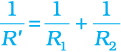and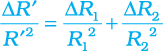The equivalent resistance of series combination

R =R1 + R2 = (100 ± 3) ohm + (200 ± 4) ohm

= 300 ± 7 ohm.

(b) The equivalent resistance of parallel combination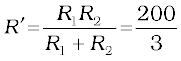= 66.7 ohm

Then, from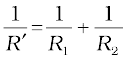we get,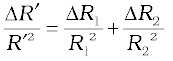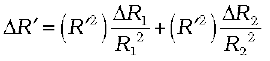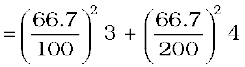= 1.8

Then,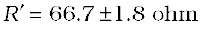(Here, R is expresed as 1.8 instead of 2 to keep in confirmity with the rules of significant figures.) t

(c) Error in case of a measured quantity raised to a power

Suppose Z = A2,

Then,

Z/Z = (A/A) + (A/A) = 2 (A/A). Hence, the relative error in A2 is two times the error in A.

In general, if Z = Ap Bq/Cr

Then,

Z/Z = p (A/A) + q (B/B) + r (C/C).

Hence the rule : The relative error in a physical quantity raised to the power k is the k times the relative error in the individual quantity.

Example 2.11 Find the relative error in Z, if Z = A4B1/3/CD3/2.

Answer The relative error in Z is Z/Z = 4(A/A) +(1/3) (B/B) + (C/C) + (3/2) (D/D).

Example 2.12 The period of oscillation of a simple pendulum is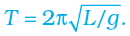Measured value of L is 20.0 cm known to 1 mm accuracy and time for 100 oscillations of the pendulum is found to be 90 s using a wrist watch of 1 s resolution. What is the accuracy in the determination of g ?

g = 4π2L/T2

Here, $T=\frac{t}{n}$ and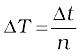. Therefore,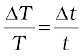. The errors in both L and t are the least count errors. Therefore,

(g/g) = (L/L) + 2(T/T )

=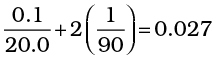Thus, the percentage error in g is

100 (g/g) = 100(L/L) + 2 × 100 (T/T )

= 3%

## 2.7 Significant figures

As discussed above, every measurement involves errors. Thus, the result of measurement should be reported in a way that indicates the precision of measurement. Normally, the reported result of measurement is a number that includes all digits in the number that are known reliably plus the first digit that is uncertain. The reliable digits plus the first uncertain digit are known as significant digits or significant figures. If we say the period of oscillation of a simple pendulum is 1.62 s, the digits 1 and 6 are reliable and certain, while the digit 2 is uncertain. Thus, the measured value has three significant figures. The length of an object reported after measurement to be 287.5 cm has four significant figures, the digits 2, 8, 7 are certain while the digit 5 is uncertain. Clearly, reporting the result of measurement that includes more digits than the significant digits is superfluous and also misleading since it would give a wrong idea about the precision of measurement.

The rules for determining the number of significant figures can be understood from the following examples. Significant figures indicate, as already mentioned, the precision of measurement which depends on the least count of the measuring instrument. A choice of change of different units does not change the number of significant digits or figures in a measurement. This important remark makes most of the following observations clear:

(1) For example, the length 2.308 cm has four significant figures. But in different units, the same value can be written as 0.02308 m or 23.08 mm or 23080 µm.

All these numbers have the same number of significant figures (digits 2, 3, 0, 8), namely four. This shows that the location of decimal point is of no consequence in determining the number of significant figures.

The example gives the following rules :

All the non-zero digits are significant.

All the zeros between two non-zero digits are significant, no matter where the decimal point is, if at all.

If the number is less than 1, the zero(s) on the right of decimal point but to the left of the first non-zero digit are not significant. [In 0.00 2308, the underlined zeroes are not significant].

The terminal or trailing zero(s) in a number without a decimal point are not significant.

[Thus 123 m = 12300 cm = 123000 mm has three significant figures, the trailing zero(s) being not significant.] However, you can also see the next observation.

The trailing zero(s) in a number with a decimal point are significant.

[The numbers 3.500 or 0.06900 have four significant figures each.]

(2) There can be some confusion regarding the trailing zero(s). Suppose a length is reported to be 4.700 m. It is evident that the zeroes here are meant to convey the precision of measurement and are, therefore, significant. [If these were not, it would be superfluous to write them explicitly, the reported measurement would have been simply 4.7 m]. Now suppose we change units, then

4.700 m = 470.0 cm = 4700 mm = 0.004700 km

Since the last number has trailing zero(s) in a number with no decimal, we would conclude erroneously from observation (1) above that the number has two significant figures, while in fact, it has four significant figures and a mere change of units cannot change the number of significant figures.

(3) To remove such ambiguities in determining the number of significant figures, the best way is to report every measurement in scientific notation (in the power of 10). In this notation, every number is expressed as a × 10b, where a is a number between 1 and 10, and b is any positive or negative exponent (or power) of 10. In order to get an approximate idea of the number, we may round off the number a to 1 (for ≤ 5) and to 10 (for 5<a ≤ 10). Then the number can be expressed approximately as 10b in which the exponent (or power) b of 10 is called order of magnitude of the physical quantity. When only an estimate is required, the quantity is of the order of 10b. For example, the diameter of the earth (1.28×107m) is of the order of 107m with the order of magnitude 7. The diameter of hydrogen atom (1.06 ×10–10m) is of the order of 10–10m, with the order of magnitude –10. Thus, the diameter of the earth is 17 orders of magnitude larger than the hydrogen atom.

It is often customary to write the decimal after the first digit. Now the confusion mentioned in (a) above disappears :

4.700 m = 4.700 × 102 cm

= 4.700 × 103 mm = 4.700 × 10–3 km

The power of 10 is irrelevant to the determination of significant figures. However, all zeroes appearing in the base number in the scientific notation are significant. Each number in this case has four significant figures.

Thus, in the scientific notation, no confusion arises about the trailing zero(s) in the base number a. They are always significant.

(4) The scientific notation is ideal for reporting measurement. But if this is not adopted, we use the rules adopted in the preceding example :

For a number greater than 1, without any decimal, the trailing zero(s) are not significant.

For a number with a decimal, the trailing zero(s) are significant.

(5) The digit 0 conventionally put on the left of a decimal for a number less than 1 (like 0.1250) is never significant. However, the zeroes at the end of such number are significant in a measurement.

(6) The multiplying or dividing factors which are neither rounded numbers nor numbers representing measured values are exact and have infinite number of significant digits. For example in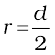or s = 2πr, the factor 2 is an exact number and it can be written as 2.0, 2.00 or 2.0000 as required. Similarly, in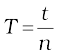, n is an exact number.

### 2.7.1 Rules for Arithmetic Operations with Significant Figures

The result of a calculation involving approximate measured values of quantities (i.e. values with limited number of significant figures) must reflect the uncertainties in the original measured values. It cannot be more accurate than the original measured values themselves on which the result is based. In general, the final result should not have more significant figures than the original data from which it was obtained. Thus, if mass of an object is measured to be, say, 4.237 g (four significant figures) and its volume is measured to be 2.51 cm3, then its density, by mere arithmetic division, is 1.68804780876 g/cm3 upto 11 decimal places. It would be clearly absurd and irrelevant to record the calculated value of density to such a precision when the measurements on which the value is based, have much less precision. The following rules for arithmetic operations with significant figures ensure that the final result of a calculation is shown with the precision that is consistent with the precision of the input measured values :

(1) In multiplication or division, the final result should retain as many significant figures as are there in the original number with the least significant figures.

Thus, in the example above, density should be reported to three significant figures.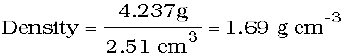Similarly, if the speed of light is given as
3
× 108 m s-1 (one significant figure) and one year (1y = 365.25 d) has 3.1557 × 107 s (five significant figures), the light year is 9.47 × 1015 m (three significant figures).

(2) In addition or subtraction, the final result should retain as many decimal places as are there in the number with the least decimal places.

For example, the sum of the numbers 436.32 g, 227.2 g and 0.301 g by mere arithmetic addition, is 663.821 g. But the least precise measurement (227.2 g) is correct to only one decimal place. The final result should, therefore, be rounded off to 663.8 g.

Similarly, the difference in length can be expressed as :

0.307 m – 0.304 m = 0.003 m = 3 × 10–3 m.

Note that we should not use the rule (1) applicable for multiplication and division and write 664 g as the result in the example of addition and 3.00 × 10–3 m in the example of subtraction. They do not convey the precision of measurement properly. For addition and subtraction, the rule is in terms of decimal places.

### 2.7.2 Rounding off the Uncertain Digits

The result of computation with approximate numbers, which contain more than one uncertain digit, should be rounded off. The rules for rounding off numbers to the appropriate significant figures are obvious in most cases. A number 2.746 rounded off to three significant figures is 2.75, while the number 2.743 would be 2.74. The rule by convention is that the preceding digit is raised by 1 if the insignificant digit to be dropped (the underlined digit in this case) is more than 5, and is left unchanged if the latter is less than 5. But what if the number is 2.745 in which the insignificant digit is 5. Here, the convention is that if the preceding digit is even, the insignificant digit is simply dropped and, if it is odd, the preceding digit is raised by 1. Then, the number 2.745 rounded off to three significant figures becomes 2.74. On the other hand, the number 2.735 rounded off to three significant figures becomes 2.74 since the preceding digit is odd.

In any involved or complex multi-step calculation, you should retain, in intermediate steps, one digit more than the significant digits and round off to proper significant figures at the end of the calculation. Similarly, a number known to be within many significant figures, such as in 2.99792458 × 108 m/s for the speed of light in vacuum, is rounded off to an approximate value 3 × 108 m/s , which is often employed in computations. Finally, remember that exact numbers that appear in formulae like 2 π in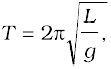have a large (infinite) number of significant figures. The value of π = 3.1415926.... is known to a large number of significant figures. You may take the value as 3.142 or 3.14 for π, with limited number of significant figures as required in specific cases.

Example 2.13 Each side of a cube is measured to be 7.203 m. What are the total surface area and the volume of the cube to appropriate significant figures?

Answer The number of significant figures in the measured length is 4. The calculated area and the volume should therefore be rounded off to 4 significant figures.

Surface area of the cube = 6(7.203)2 m2

= 311.299254 m2

= 311.3 m2

Volume of the cube = (7.203)3 m3

= 373.714754 m3

= 373.7 m3

Example 2.14 5.74 g of a substance occupies 1.2 cm3. Express its density by keeping the significant figures in view.

Answer There are 3 significant figures in the measured mass whereas there are only 2 significant figures in the measured volume. Hence the density should be expressed to only 2 significant figures.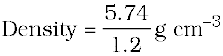= 4.8 g cm-3 .

### 2.7.3 Rules for Determining the Uncertainty in the Results of Arithmatic Calculations

The rules for determining the uncertainty or error in the number/measured quantity in arithmetic operations can be understood from the following examples.

(1) If the length and breadth of a thin rectangular sheet are measured, using a metre scale as 16.2 cm and, 10.1 cm respectively, there are three significant figures in each measurement. It means that the length l may be written as

l = 16.2 ± 0.1 cm

= 16.2 cm ± 0.6 %.

Similarly, the breadth b may be written as

b = 10.1 ± 0.1 cm

= 10.1 cm ± 1 %

Then, the error of the product of two (or more) experimental values, using the combination of errors rule, will be

l b = 163.62 cm2 + 1.6%

= 163.62 + 2.6 cm2

This leads us to quote the final result as

l b = 164 + 3 cm2

Here 3 cm2 is the uncertainty or error in the estimation of area of rectangular sheet.

(2) If a set of experimental data is specified to n significant figures, a result obtained by combining the data will also be valid to n significant figures.

However, if data are subtracted, the number of significant figures can be reduced.

For example, 12.9 g – 7.06 g, both specified to three significant figures, cannot properly be evaluated as 5.84 g but only as 5.8 g, as uncertainties in subtraction or addition combine in a different fashion (smallest number of decimal places rather than the number of significant figures in any of the number added or subtracted).

(3) The relative error of a value of number specified to significant figures depends not only on n but also on the number itself.

For example, the accuracy in measurement of mass 1.02 g is ± 0.01 g whereas another measurement 9.89 g is also accurate to ± 0.01 g.

The relative error in 1.02 g is

= (± 0.01/1.02) × 100 %

= ± 1%

Similarly, the relative error in 9.89 g is

= (± 0.01/9.89) × 100 %

= ± 0.1 %

Finally, remember that intermediate results in a multi-step computation should be calculated to one more significant figure in every measurement than the number of digits in the least precise measurement. These should be justified by the data and then the arithmetic operations may be carried out; otherwise rounding errors can build up. For example, the reciprocal of 9.58, calculated (after rounding off) to the same number of significant figures (three) is 0.104, but the reciprocal of 0.104 calculated to three significant figures is 9.62. However, if we had written 1/9.58 = 0.1044 and then taken the reciprocal to three significant figures, we would have retrieved the original value of 9.58.

This example justifies the idea to retain one more extra digit (than the number of digits in the least precise measurement) in intermediate steps of the complex multi-step calculations in order to avoid additional errors in the process of rounding off the numbers.

## 2.8Dimensions of physical quantities

The nature of a physical quantity is described by its dimensions. All the physical quantities represented by derived units can be expressed in terms of some combination of seven fundamental or base quantities. We shall call these base quantities as the seven dimensions of the physical world, which are denoted with square brackets [ ]. Thus, length has the dimension [L], mass [M], time [T], electric current [A], thermodynamic temperature [K], luminous intensity [cd], and amount of substance [mol]. The dimensions of a physical quantity are the powers (or exponents) to which the base quantities are raised to represent that quantity. Note that using the square brackets [ ] round a quantity means that we are dealing with ‘the dimensions ofthe quantity.

In mechanics, all the physical quantities can be written in terms of the dimensions [L], [M] and [T]. For example, the volume occupied by an object is expressed as the product of length, breadth and height, or three lengths. Hence the dimensions of volume are [L] × [L] × [L] = [L]3 = [L3]. As the volume is independent of mass and time, it is said to possess zero dimension in mass [M°], zero dimension in time [T°] and three dimensions in length.

Similarly, force, as the product of mass and acceleration, can be expressed as

Force = mass × acceleration

= mass × (length)/(time)2

The dimensions of force are [M] [L]/[T]2 = [M L T–2]. Thus, the force has one dimension in mass, one dimension in length, and –2 dimensions in time. The dimensions in all other base quantities are zero.

Note that in this type of representation, the magnitudes are not considered. It is the quality of the type of the physical quantity that enters. Thus, a change in velocity, initial velocity, average velocity, final velocity, and speed are all equivalent in this context. Since all these quantities can be expressed as length/time, their dimensions are [L]/[T] or [L T–1].

## 2.9 Dimensional formulae and dimensional equations

The expression which shows how and which of the base quantities represent the dimensions of a physical quantity is called the dimensional formula of the given physical quantity. For example, the dimensional formula of the volume is [M° L3 T°], and that of speed or velocity is
[M° L T
-1]. Similarly, [M° L T–2] is the dimensional formula of acceleration and [M L–3 T°] that of mass density.

An equation obtained by equating a physical quantity with its dimensional formula is called the dimensional equation of the physical quantity. Thus, the dimensional equations are the equations, which represent the dimensions of a physical quantity in terms of the base quantities. For example, the dimensional equations of volume [V], speed [v], force [F] and mass density [ρ] may be expressed as

[V] = [M0 L3 T0]

[v] = [M0 L T–1]

[F] = [M L T–2]

[ρ] = [M L–3 T0]

The dimensional equation can be obtained from the equation representing the relations between the physical quantities. The dimensional formulae of a large number and wide variety of physical quantities, derived from the equations representing the relationships among other physical quantities and expressed in terms of base quantities are given in Appendix 9 for your guidance and ready reference.

## 2.10 Dimensional analysis and its applications

The recognition of concepts of dimensions, which guide the description of physical behaviour is of basic importance as only those physical quantities can be added or subtracted which have the same dimensions. A thorough understanding of dimensional analysis helps us in deducing certain relations among different physical quantities and checking the derivation, accuracy and dimensional consistency or homogeneity of various mathematical expressions. When magnitudes of two or more physical quantities are multiplied, their units should be treated in the same manner as ordinary algebraic symbols. We can cancel identical units in the numerator and denominator. The same is true for dimensions of a physical quantity. Similarly, physical quantities represented by symbols on both sides of a mathematical equation must have the same dimensions.

### 2.10.1 Checking the Dimensional Consistency of Equations

The magnitudes of physical quantities may be added together or subtracted from one another only if they have the same dimensions. In other words, we can add or subtract similar physical quantities. Thus, velocity cannot be added to force, or an electric current cannot be subtracted from the thermodynamic temperature. This simple principle called the principle of homogeneity of dimensions in an equation is extremely useful in checking the correctness of an equation. If the dimensions of all the terms are not same, the equation is wrong. Hence, if we derive an expression for the length (or distance) of an object, regardless of the symbols appearing in the original mathematical relation, when all the individual dimensions are simplified, the remaining dimension must be that of length. Similarly, if we derive an equation of speed, the dimensions on both the sides of equation, when simplified, must be of length/time, or [L T–1].

Dimensions are customarily used as a preliminary test of the consistency of an equation, when there is some doubt about the correctness of the equation. However, the dimensional consistency does not guarantee correct equations. It is uncertain to the extent of dimensionless quantities or functions. The arguments of special functions, such as the trigonometric, logarithmic and exponential functions must be dimensionless. A pure number, ratio of similar physical quantities, such as angle as the ratio (length/length), refractive index as the ratio (speed of light in vacuum/speed of light in medium) etc., has no dimensions.

Now we can test the dimensional consistency or homogeneity of the equation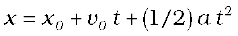for the distance x travelled by a particle or body in time t which starts from the position x0 with an initial velocity v0 at time t = 0 and has uniform acceleration a along the direction of motion.

The dimensions of each term may be written as

[x] = [L]

[x0 ] = [L]

[v0 t] = [L T–1] [T]

= [L]

[(1/2) a t2] = [L T–2] [T2]

= [L]

As each term on the right hand side of this equation has the same dimension, namely that of length, which is same as the dimension of left hand side of the equation, hence this equation is a dimensionally correct equation.

It may be noted that a test of consistency of dimensions tells us no more and no less than a test of consistency of units, but has the advantage that we need not commit ourselves to a particular choice of units, and we need not worry about conversions among multiples and sub-multiples of the units. It may be borne in mind that if an equation fails this consistency test, it is proved wrong, but if it passes, it is not proved right. Thus, a dimensionally correct equation need not be actually an exact (correct) equation, but a dimensionally wrong (incorrect) or inconsistent equation must be wrong.

Example 2.15 Let us consider an equation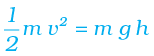where m is the mass of the body, v its velocity, g is the acceleration due to gravity and h is the height. Check whether this equation is dimensionally correct.

Answer The dimensions of LHS are

[M] [L T–1 ]2 = [M] [ L2 T–2]

= [M L2 T–2]

The dimensions of RHS are

[M][L T–2] [L] = [M][L2 T–2]

= [M L2 T–2]

The dimensions of LHS and RHS are the same and hence the equation is dimensionally correct.

Example 2.16 The SI unit of energy is J = kg m2 s–2; that of speed v is m s–1 and of acceleration a is m s–2. Which of the formulae for kinetic energy (K) given below can you rule out on the basis of dimensional arguments (m stands for the mass of the body) :

(a) K = m2 v3

(b) K = (1/2)mv2

(c) K = ma

(d) K = (3/16)mv2

(e) K = (1/2)mv2 + ma

Answer   Every correct formula or equation must have the same dimensions on both sides of the equation. Also, only quantities with the same physical dimensions can be added or subtracted. The dimensions of the quantity on the right side are [M2 L3 T–3] for (a); [M L2 T–2] for (b) and (d); [M L T–2] for (c). The quantity on the right side of (e) has no proper dimensions since two quantities of different dimensions have been added. Since the kinetic energy K has the dimensions of [M L2 T–2], formulas (a), (c) and (e) are ruled out. Note that dimensional arguments cannot tell which of the two, (b) or (d), is the correct formula. For this, one must turn to the actual definition of kinetic energy (see Chapter 6). The correct formula for kinetic energy is given by (b).

### 2.10.2 Deducing Relation among the Physical Quantities

The method of dimensions can sometimes be used to deduce relation among the physical quantities. For this we should know the dependence of the physical quantity on other quantities (upto three physical quantities or linearly independent variables) and consider it as a product type of the dependence. Let us take an example.

Example 2.17 Consider a simple pendulum, having a bob attached to a string, that oscillates under the action of the force of gravity. Suppose that the period of oscillation of the simple pendulum depends on its length (l), mass of the bob (m) and acceleration due to gravity (g). Derive the expression for its time period using method of dimensions.

Answer The dependence of time period T on the quantities l, g and m as a product may be written as :

T = k lx gy mz

where k is dimensionless constant and x, y and z are the exponents.

By considering dimensions on both sides, we have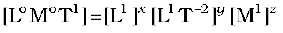= Lx+y T–2y Mz

On equating the dimensions on both sides, we have

x + y = 0; –2y = 1; and z = 0

So that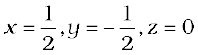Then, T = k l½ g½

or, T =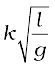Note that value of constant k can not be obtained by the method of dimensions. Here it does not matter if some number multiplies the right side of this formula, because that does not affect its dimensions.

Actually, k = 2π so that T =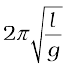Dimensional analysis is very useful in deducing relations among the interdependent physical quantities. However, dimensionless constants cannot be obtained by this method. The method of dimensions can only test the dimensional validity, but not the exact relationship between physical quantities in any equation. It does not distinguish between the physical quantities having same dimensions.

A number of exercises at the end of this chapter will help you develop skill in dimensional analysis.

## Summary

1. Physics is a quantitative science, based on measurement of physical quantities. Certain physical quantities have been chosen as fundamental or base quantities (such as length, mass, time, electric current, thermodynamic temperature, amount of substance, and luminous intensity).

2. Each base quantity is defined in terms of a certain basic, arbitrarily chosen but properly standardised reference standard called unit (such as metre, kilogram, second, ampere, kelvin, mole and candela). The units for the fundamental or base quantities are called fundamental or base units.

3. Other physical quantities, derived from the base quantities, can be expressed as a combination of the base units and are called derived units. A complete set of units, both fundamental and derived, is called a system of units.

4. The International System of Units (SI) based on seven base units is at present internationally accepted unit system and is widely used throughout the world.

5. The SI units are used in all physical measurements, for both the base quantities and the derived quantities obtained from them. Certain derived units are expressed by means of SI units with special names (such as joule, newton, watt, etc).

6. The SI units have well defined and internationally accepted unit symbols (such as m for metre, kg for kilogram, s for second, A for ampere, N for newton etc.).

7. Physical measurements are usually expressed for small and large quantities in scientific notation, with powers of 10. Scientific notation and the prefixes are used to simplify measurement notation and numerical computation, giving indication to the precision of the numbers.

8. Certain general rules and guidelines must be followed for using notations for physical quantities and standard symbols for SI units, some other units and SI prefixes for expressing properly the physical quantities and measurements.

9. In computing any physical quantity, the units for derived quantities involved in the relationship(s) are treated as though they were algebraic quantities till the desired units are obtained.

10. Direct and indirect methods can be used for the measurement of physical quantities. In measured quantities, while expressing the result, the accuracy and precision of measuring instruments along with errors in measurements should be taken into account.

11. In measured and computed quantities proper significant figures only should be retained. Rules for determining the number of significant figures, carrying out arithmetic operations with them, and ‘rounding off ‘ the uncertain digits must be followed.

12. The dimensions of base quantities and combination of these dimensions describe the nature of physical quantities. Dimensional analysis can be used to check the dimensional consistency of equations, deducing relations among the physical quantities, etc. A dimensionally consistent equation need not be actually an exact (correct) equation, but a dimensionally wrong or inconsistent equation must be wrong.

## Exercises

##### Note : In stating numerical answers, take care of significant figures.

2.1 Fill in the blanks

(a) The volume of a cube of side 1 cm is equal to .....m3

(b) The surface area of a solid cylinder of radius 2.0 cm and height 10.0 cm is equal to...(mm)2

(c) A vehicle moving with a speed of 18 km h–1 covers....m in 1 s

(d) The relative density of lead is 11.3. Its density is ....g cm–3 or ....kg m–3.

2.2 Fill in the blanks by suitable conversion of units

(a) 1 kg m2 s–2 = ....g cm2 s–2

(b) 1 m = ..... ly

(c) 3.0 m s–2 = .... km h–2

(d) G = 6.67 × 10–11 N m2 (kg)–2 = .... (cm)3 s–2 g–1.

2.3 A calorie is a unit of heat (energy in transit) and it equals about 4.2 J where 1J = 1 kg m2 s–2. Suppose we employ a system of units in which the unit of mass equals α kg, the unit of length equals β m, the unit of time is γ s. Show that a calorie has a magnitude 4.2 α–1 β–2 γ2 in terms of the new units.

2.4 Explain this statement clearly :

“To call a dimensional quantity ‘large’ or ‘small’ is meaningless without specifying a standard for comparison”. In view of this, reframe the following statements wherever necessary :

(a) atoms are very small objects

(b) a jet plane moves with great speed

(c) the mass of Jupiter is very large

(d) the air inside this room contains a large number of molecules

(e) a proton is much more massive than an electron

(f) the speed of sound is much smaller than the speed of light.

2.5 A new unit of length is chosen such that the speed of light in vacuum is unity. What is the distance between the Sun and the Earth in terms of the new unit if light takes 8 min and 20 s to cover this distance ?

2.6 Which of the following is the most precise device for measuring length :

(a) a vernier callipers with 20 divisions on the sliding scale

(b) a screw gauge of pitch 1 mm and 100 divisions on the circular scale

(c) an optical instrument that can measure length to within a wavelength of light ?

2.7 A student measures the thickness of a human hair by looking at it through a microscope of magnification 100. He makes 20 observations and finds that the average width of the hair in the field of view of the microscope is 3.5 mm. What is the estimate on the thickness of hair ?

(a) You are given a thread and a metre scale. How will you estimate the diameter of the thread ?

(b) A screw gauge has a pitch of 1.0 mm and 200 divisions on the circular scale. Do you think it is possible to increase the accuracy of the screw gauge arbitrarily by increasing the number of divisions on the circular scale ?

(c) The mean diameter of a thin brass rod is to be measured by vernier callipers. Why is a set of 100 measurements of the diameter expected to yield a more reliable estimate than a set of 5 measurements only ?

2.9 The photograph of a house occupies an area of 1.75 cm2 on a 35 mm slide. The slide is projected on to a screen, and the area of the house on the screen is 1.55 m2. What is the linear magnification of the projector-screen arrangement.

2.10 State the number of significant figures in the following :

(a) 0.007 m2

(b) 2.64 × 1024 kg

(c) 0.2370 g cm–3

(d) 6.320 J

(e) 6.032 N m–2

(f) 0.0006032 m2

2.11 The length, breadth and thickness of a rectangular sheet of metal are 4.234 m, 1.005 m, and 2.01 cm respectively. Give the area and volume of the sheet to correct significant figures.

2.12 The mass of a box measured by a grocer’s balance is 2.30 kg. Two gold pieces of masses 20.15 g and 20.17 g are added to the box. What is (a) the total mass of the box, (b) the difference in the masses of the pieces to correct significant figures ?

2.13 A physical quantity P is related to four observables a, b, c and d as follows :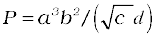The percentage errors of measurement in a, b, c and d are 1%, 3%, 4% and 2%, respectively. What is the percentage error in the quantity P ? If the value of P calculated using the above relation turns out to be 3.763, to what value should you round off the result ?

2.14 A book with many printing errors contains four different formulas for the displacement y of a particle undergoing a certain periodic motion :

(a) y = a sin 2π t/T

(b) y = a sin vt

(c) y = (a/T) sin t/a

(d)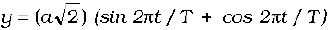(a = maximum displacement of the particle, v = speed of the particle. T = time-period of motion). Rule out the wrong formulas on dimensional grounds.

2.15 A famous relation in physics relates ‘moving mass’ m to the ‘rest mass’ mo of a particle in terms of its speed v and the speed of light, c. (This relation first arose as a consequence of special relativity due to Albert Einstein). A boy recalls the relation almost correctly but forgets where to put the constant c. He writes :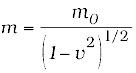.

Guess where to put the missing c.

2.16 The unit of length convenient on the atomic scale is known as an angstrom and is denoted by Å: 1 Å = 10–10 m. The size of a hydrogen atom is about 0.5 Å. What is the total atomic volume in m3 of a mole of hydrogen atoms ?

2.17 One mole of an ideal gas at standard temperature and pressure occupies 22.4 L (molar volume). What is the ratio of molar volume to the atomic volume of a mole of hydrogen ? (Take the size of hydrogen molecule to be about 1 Å). Why is this ratio so large ?

2.18 Explain this common observation clearly : If you look out of the window of a fast moving train, the nearby trees, houses etc. seem to move rapidly in a direction opposite to the train’s motion, but the distant objects (hill tops, the Moon, the stars etc.) seem to be stationary. (In fact, since you are aware that you are moving, these distant objects seem to move with you).

2.19 The principle of ‘parallax’ in section 2.3.1 is used in the determination of distances of very distant stars. The baseline AB is the line joining the Earth’s two locations six months apart in its orbit around the Sun. That is, the baseline is about the diameter of the Earth’s orbit 3 × 1011m. However, even the nearest stars are so distant that with such a long baseline, they show parallax only of the order of 1” (second) of arc or so. A parsec is a convenient unit of length on the astronomical scale. It is the distance of an object that will show a parallax of 1” (second of arc) from opposite ends of a baseline equal to the distance from the Earth to the Sun. How much is a parsec in terms of metres ?

2.20 The nearest star to our solar system is 4.29 light years away. How much is this distance in terms of parsecs? How much parallax would this star (named Alpha Centauri) show when viewed from two locations of the Earth six months apart in its orbit around the Sun ?

2.21 Precise measurements of physical quantities are a need of science. For example, to ascertain the speed of an aircraft, one must have an accurate method to find its positions at closely separated instants of time. This was the actual motivation behind the discovery of radar in World War II. Think of different examples in modern science where precise measurements of length, time, mass etc. are needed. Also, wherever you can, give a quantitative idea of the precision needed.

2.22 Just as precise measurements are necessary in science, it is equally important to be able to make rough estimates of quantities using rudimentary ideas and common observations. Think of ways by which you can estimate the following (where an estimate is difficult to obtain, try to get an upper bound on the quantity) :

(a) the total mass of rain-bearing clouds over India during the Monsoon

(b) the mass of an elephant

(c) the wind speed during a storm

(e) the number of air molecules in your classroom.

2.23 The Sun is a hot plasma (ionized matter) with its inner core at a temperature exceeding 107 K, and its outer surface at a temperature of about 6000 K. At these high temperatures, no substance remains in a solid or liquid phase. In what range do you expect the mass density of the Sun to be, in the range of densities of solids and liquids or gases ? Check if your guess is correct from the following data : mass of the Sun = 2.0 ×1030 kg, radius of the Sun = 7.0 × 108 m.

2.24 When the planet Jupiter is at a distance of 824.7 million kilometers from the Earth, its angular diameter is measured to be 35.72” of arc. Calculate the diameter of Jupiter.

2.25 A man walking briskly in rain with speed v must slant his umbrella forward making an angle θ with the vertical. A student derives the following relation between θ and v : tan θ = v and checks that the relation has a correct limit: as v 0, θ 0, as expected. (We are assuming there is no strong wind and that the rain falls vertically for a stationary man). Do you think this relation can be correct ? If not, guess the correct relation.

2.26 It is claimed that two cesium clocks, if allowed to run for 100 years, free from any disturbance, may differ by only about 0.02 s. What does this imply for the accuracy of the standard cesium clock in measuring a time-interval of 1 s ?

2.27 Estimate the average mass density of a sodium atom assuming its size to be about 2.5 Å. (Use the known values of Avogadro’s number and the atomic mass of sodium). Compare it with the mass density of sodium in its crystalline phase : 970 kg m–3. Are the two densities of the same order of magnitude ? If so, why ?

2.28 The unit of length convenient on the nuclear scale is a fermi : 1 f = 10–15 m. Nuclear sizes obey roughly the following empirical relation :

r = r0 A1/3

where r is the radius of the nucleus, A its mass number, and ro is a constant equal to about, 1.2 f. Show that the rule implies that nuclear mass density is nearly constant for different nuclei. Estimate the mass density of sodium nucleus. Compare it with the average mass density of a sodium atom obtained in Exercise. 2.27.

2.29 A LASER is a source of very intense, monochromatic, and unidirectional beam of light. These properties of a laser light can be exploited to measure long distances. The distance of the Moon from the Earth has been already determined very precisely using a laser as a source of light. A laser light beamed at the Moon takes 2.56 s to return after reflection at the Moon’s surface. How much is the radius of the lunar orbit around the Earth ?

2.30 A SONAR (sound navigation and ranging) uses ultrasonic waves to detect and locate objects under water. In a submarine equipped with a SONAR, the time delay between generation of a probe wave and the reception of its echo after reflection from an enemy submarine is found to be 77.0 s. What is the distance of the enemy submarine? (Speed of sound in water = 1450 m s–1).

2.31 The farthest objects in our Universe discovered by modern astronomers are so distant that light emitted by them takes billions of years to reach the Earth. These objects (known as quasars) have many puzzling features, which have not yet been satisfactorily explained. What is the distance in km of a quasar from which light takes 3.0 billion years to reach us?

2.32 It is a well known fact that during a total solar eclipse the disk of the moon almost completely covers the disk of the Sun. From this fact and from the information you can gather from examples 2.3 and 2.4, determine the approximate diameter of the moon.

2.33 A great physicist of this century (P.A.M. Dirac) loved playing with numerical values of Fundamental constants of nature. This led him to an interesting observation. Dirac found that from the basic constants of atomic physics (c, e, mass of electron, mass of proton) and the gravitational constant G, he could arrive at a number with the dimension of time. Further, it was a very large number, its magnitude being close to the present estimate on the age of the universe (~15 billion years). From the table of fundamental constants in this book, try to see if you too can construct this number (or any other interesting number you can think of ). If its coincidence with the age of the universe were significant, what would this imply for the constancy of fundamental constants?

Chapter 2

2.1 (a) 10–6 ; (b) 1.5 × 104 ; (c) 5 ; (d) 11.3, 1.13 × 104.

2.2 (a) 107 ; (b) 10–16 ; (c) 3.9 × 104 ; (d) 6.67 × 10–8.

2.5 500

2.6 (c)

2.7 0.035 mm

2.9 94.1

2.10 (a) 1 ; (b) 3 ; (c) 4 ; (d) 4 ; (e) 4 ; (f) 4.

2.11 8.72 m2; 0.0855 m3

2.12 (a) 2.340 kg ; (b) 0.02 g

2.13 13%; 3.8

2.14 (b) and (c) are wrong on dimensional grounds. Hint: The argument of a trigonometric function must always be dimensionless.

2.15 The correct formula is m = m0 (1 – v2/c2)–½

2.16 3 × 10–7 m3

2.17 104; intermolecular separation in a gas is much larger than the size of a molecule.

2.18 Near objects make greater angle than distant (far off) objects at the eye of the observer. When you are moving, the angular change is less for distant objects than nearer objects. So, these distant objects seem to move along with you, but the nearer object in opposite direction.

2.19 3 × 1016 m; as a unit of length 1 parsec is defined to be equal to 3.084 × 1016 m.

2.20 1.32 parsec; 2.64 (second of arc)

2.23 1.4 × 103 kg m-3; the mass density of the Sun is in the range of densities of liquids /solids and not gases. This high density arises due to inward gravitational attraction on outer layers due to inner layers of the Sun.

2.24 1.429 × 105 km

2.25 Hint: tan θ must be dimensionless. The correct formula is tan θ = v/v where v is the speed of rainfall.

2.26 Accuracy of 1 part in 1011 to 1012

2.27 4.6 × 103 kg m–3. In the solid phase atoms are tightly packed, so the atomic mass density is close to the mass density of the solid.

2.28 4.7 × 1018 m–3 – Nuclear density is typically 1015 times atomic density of matter.

2.29 3.84 × 108 m

2.30 55.8 km

2.31 2.8 × 1022 km

2.32 3,581 km

2.33 Hint: the quantity e4/ (16 π220 mp me2 c3 G ) has the dimension of time.

## Exercises

##### Note: In stating numerical answers, take care of significant figures.

2.1 Fill in the blanks.

(a) The volume of a cube of side 1 cm is equal to .....m3

(b) The surface area of a solid cylinder of radius 2.0 cm and height 10.0 cm is equal to .....(mm)2

(c) A vehicle moving with a speed of 18 km h–1 covers ......m in 1 s

(d) The relative density of lead is 11.3. Its density is ....g cm–3 or ....kg m–3.

2.2 Fill in the blanks by suitable conversion of units.

(a) 1 kg m2 s–2 = ....g cm2 s–2

(b) 1 m = ..... ly

(c) 3.0 m s–2 = .... km h–2

(d) G = 6.67 × 10–11 N m2 (kg)–2 = .... (cm)3 s–2 g–1.

2.3 A calorie is a unit of heat (energy in transit) and it equals about 4.2 J where 1 J = 1 kgm2s–2. Suppose we employ a system of units in which the unit of mass equals α kg, the unit of length equals β m, the unit of time is γ sec. Show that a calorie has a magnitude of 4.2 α–1β–2γ2 in terms of the new units.

2.4 Explain this statement clearly :

“To call a dimensional quantity ‘large’ or ‘small’ is meaningless without specifying a standard for comparison”. In view of this, reframe the following statements wherever necessary :

(a) Atoms are very small objects

(b) a jet plane moves with great speed

(c) the mass of Jupiter is very large

(d) the air inside this room contains a large number of molecules

(e) a proton is much more massive than an electron

(f) the speed of sound is much smaller than the speed of light.

2.5 A new unit of length is chosen such that the speed of light in a vacuum is unity. What is the distance between the Sun and the Earth in terms of the new unit if light takes 8 min and 20 s to cover this distance?

2.6 Which of the following is the most precise device for measuring length?

(a) A vernier callipers with 20 divisions on the sliding scale.

(b) A screw gauge of pitch 1 mm and 100 divisions on the circular scale

(c) An optical instrument that can measure the length to within a wavelength of light.

(d) Both (a) and (b)

2.7 A student measures the thickness of a human hair by looking at it through a microscope of magnification 100. He makes 20 observations and finds that the average width of the hair in the field of view of the microscope is 3.5 mm. What is the estimate of the thickness of the hair?

(a) You are given a thread and a meter scale. How will you estimate the diameter of the thread?

(b) A screw gauge has a pitch of 1.0 mm and 200 divisions on the circular scale. Do you think it is possible to increase the accuracy of the screw gauge arbitrarily by increasing the number of divisions on the circular scale?

(c) The mean diameter of a thin brass rod is to be measured by vernier callipers. Why is a set of 100 measurements of the diameter expected to yield a more reliable estimate than a set of 5 measurements only?

2.9 The photograph of a house occupies an area of 1.75 cm2 on a 35 mm slide. The slide is projected onto a screen, and the area of the house on the screen is 1.55 m2. What is the linear magnification of the projector-screen arrangement?

2.10 State the number of significant figures in the following :

(a) 0.007 m2

(b) 2.64 × 1024 kg

(c) 0.2370 g cm–3

(d) 6.320 J

(e) 6.032 N m–2

(f) 0.0006032 m2

## Exercises

2.11 The length, breadth, and thickness of a rectangular sheet of metal are 4.234 m, 1.005 m, and 2.01 cm respectively. Give the area and volume of the sheet to correct significant figures.

2.12 The mass of a box measured by a grocer’s balance is 2.300 kg. Two gold pieces of masses 20.15 g and 20.17 g are added to the box. What is (a) the total mass of the box, (b) the difference in the masses of the pieces to correct significant figures?

2.13 A physical quantity P is related to four observables a, b, c, and d as follows :

$P={a}^{3}{b}^{2}/\sqrt{c}d$

The percentage errors of measurement in a, b, c, and d are 1%, 3%, 4%, and 2%, respectively. What is the percentage error in the quantity P ? If the value of P calculated using the above relation turns out to be 3.763, to what value should you round off the result?

2.14 A book with many printing errors contains four different formulas for the displacement y of a particle undergoing a certain periodic motion :

(a) y = a sin 2π t/T

(b) y = a sin vt

(c) y = (a/T) sin t/a

(d)

(a = maximum displacement of the particle, v = speed of the particle. T = time-period of motion). Rule out the wrong formulas on dimensional grounds.

2.15 A famous relation in physics relates ‘moving mass m to the ‘rest mass’ ${m}_{0}$ of a particle in terms of its speed v and the speed of light, c. (This relation first arose as a consequence of special relativity due to Albert Einstein). A boy recalls the relation almost correctly but forgets where to put the constant c. He writes :

$m=\frac{{m}_{0}}{{\left(1-{v}^{2}\right)}^{1/2}}$ .

Guess where to put the missing c.

2.16 The unit of length convenient on the atomic scale is known as an angstrom and is denoted by Å: 1 Å = 10–10 m. The size of a hydrogen atom is about 0.5 Å. What is the total atomic volume in m3 of a mole of hydrogen atoms?

2.17 One mole of an ideal gas at standard temperature and pressure occupies 22.4 L (molar volume). What is the ratio of molar volume to the atomic volume of a mole of hydrogen? (Take the size of the hydrogen molecule to be about 1 Å). Why is this ratio so large?

2.18 Explain this common observation clearly: If you look out of the window of a fast-moving train, the nearby trees, houses, etc. seem to move rapidly in a direction opposite to the train’s motion, but the distant objects (hilltops, the Moon, the stars, etc.) seem to be stationary. (In fact, since you are aware that you are moving, these distant objects seem to move with you).

2.19 The principle of ‘parallax’ in section 2.3.1 is used in the determination of distances of very distant stars. The baseline AB is the line joining the Earth’s two locations six months apart in its orbit around the Sun. That is, the baseline is about the diameter of the Earth’s orbit 3 × 1011m. However, even the nearest stars are so distant that with such a long baseline, they show parallax only of the order of 1” (second) of arc or so. A parsec is a convenient unit of length on the astronomical scale. It is the distance of an object that will show a parallax of 1” (second of arc) from opposite ends of a baseline equal to the distance from the Earth to the Sun. How much is a parsec in terms of metres?

2.20 The nearest star to our solar system is 4.29 light-years away. How much is this distance in terms of parsecs? How much parallax would this star (named Alpha Centauri) show when viewed from two locations of the Earth six months apart in its orbit around the Sun?

##### Note: In stating numerical answers, take care of significant figures.

2.21 Precise measurements of physical quantities are a need of science. For example, to ascertain the speed of an aircraft, one must have an accurate method to find its positions at closely separated instants of time. This was the actual motivation behind the discovery of radar in World War II. Think of different examples in modern science where precise measurements of length, time, mass, etc. are needed. Also, wherever you can, give a quantitative idea of the precision needed.

2.22 Just as precise measurements are necessary for science, it is equally important to be able to make rough estimates of quantities using rudimentary ideas and common observations. Think of ways by which you can estimate the following (where an estimate is difficult to obtain, try to get an upper bound on the quantity) :

(a) the total mass of rain-bearing clouds over India during the Monsoon

(b) the mass of an elephant

(c) the wind speed during a storm

(e) the number of air molecules in your classroom.

2.23 The Sun is a hot plasma (ionized matter) with its inner core at a temperature exceeding 107 K, and its outer surface at a temperature of about 6000 K. At these high temperatures, no substance remains in a solid or liquid phase. In what range do you expect the mass density of the Sun to be, in the range of densities of solids and liquids or gases? Check if your guess is correct from the following data: the mass of the Sun = 2.0 ×1030 kg, the radius of the Sun = 7.0 × 108 m.

2.24 When the planet Jupiter is at a distance of 824.7 million kilometers from the Earth, its angular diameter is measured to be 35.72” of arc. Calculate the diameter of Jupiter.

Question 2.25:A man walking briskly in rain with speed v must slant his umbrella forward making an angle θ with the vertical. A student derives the following relation between θ and v: tan θ = v, and checks that the relation has a correct limit: as v → 0, θ → 0, as expected. (We are assuming there is no strong wind and that the rain falls vertically for a stationary man). Do you think this relation can be correct? If not, guess the correct relation.

Question 2.26:

It is claimed that two cesium clocks if allowed to run for 100 years, free from any disturbance, may differ by only about 0.02 s. What does this imply for the accuracy of the standard cesium clock in measuring a time-interval of 1 s?

Question 2.27:

Estimate the average mass density of a sodium atom assuming its size to be about  (Use the known values of Avogadro’s number and the atomic mass of sodium). Compare it with the density of sodium in its crystalline phase:  Are the two densities of the same order of magnitude? If so, why?

Question 2.28:

The unit of length convenient on the nuclear scale is a fermi:  Nuclear sizes obey roughly the following empirical relation:

where r is the radius of the nucleus, A its mass number, and r0 is a constant equal to about, 1.2 f. Show that the rule implies that nuclear mass density is nearly constant for different nuclei. Estimate the mass density of the sodium nucleus. Compare it with the average mass density of a sodium atom obtained in Exercise. 2.27.

Question 2.29:

A LASER is a source of very intense, monochromatic, and unidirectional beam of light. These properties of a laser light can be exploited to measure long distances. The distance of the Moon from the Earth has been already determined very precisely using a laser as a source of light. A laser light beamed at the Moon takes 2.56 s to return after reflection at the Moon’s surface. How much is the radius of the lunar orbit around the Earth?

A SONAR (sound navigation and ranging) uses ultrasonic waves to detect and locate objects underwater. In a submarine equipped with a SONAR, the time delay between the generation of a probe wave and the reception of its echo after reflection from an enemy submarine is found to be 77.0 s. What is the distance of the enemy submarine? (Speed of sound in water = 1450 m/s ).

Question 2.31:

The farthest objects in our Universe discovered by modern astronomers are so distant that light emitted by them takes billions of years to reach the Earth. These objects (known as quasars) have many puzzling features, which have not yet been satisfactorily explained. What is the distance in km of a quasar from which light takes 3.0 billion years to reach us?

Question 2.32:

It is a well-known fact that during a total solar eclipse the disk of the moon almost completely covers the disk of the Sun. From this fact and from the information you can gather from examples 2.3 and 2.4, determine the approximate diameter of the moon.

Question 2.33:

A great physicist of this century (P.A.M. Dirac) loved playing with numerical values of fundamental constants of nature. This led him to an interesting observation. Dirac found that from the basic constants of atomic physics (c, e, mass of the electron, the mass of a proton) and the gravitational constant G, he could arrive at a number with the dimension of time. Further, it was a very large number, its magnitude being close to the present estimate on the age of the universe (~15 billion years). From the table of fundamental constants in this book, try to see if you too can construct this number (or any other interesting number you can think of). If its coincidence with the age of the universe were significant, what would this imply for the constancy of fundamental constants?

EXEMPLAR QUESTIONS

The number of significant figures in 0.06900 is

(a) 5

(b) 4

(c) 2

(d) 3

The sum of the numbers 436.32, 227.2, and 0.301 inappropriate significant figures is

(a) 663.821

(b) 664

(c) 663.8

(d) 663.82

The mass and volume of a body are 4.237 g and 2.5 ${\mathrm{cm}}^{3}$, respectively. The density of the material of the body in correct significant figures is

(a) 1.6048 g ${\mathrm{cm}}^{-3}$

(b) 1.69 g ${\mathrm{cm}}^{-3}$

(c) 1.7 g ${\mathrm{cm}}^{-3}$

(d) 1.695 g ${\mathrm{cm}}^{-3}$

The numbers 2.745 and 2.735 on rounding off to 3 significant figures will give

(a) 2.75 and 2.74

(b) 2.74 and 2.73

(c) 2.75 and 2.73

(d) 2.74 and 2.74

The length and breadth of a rectangular sheet are 16.2 cm and 10.1 cm,
respectively. The area of the sheet inappropriate significant figures and error is

(a) 164 $±$ 3 ${\mathrm{cm}}^{2}$

(b) 163.62 $±$ 2.6 ${\mathrm{cm}}^{2}$

(c) 163.6 $±$ 2.6 ${\mathrm{cm}}^{2}$

(d) 163.62 $±$ 3 ${\mathrm{cm}}^{2}$

Which of the following pairs of physical quantities does not have the same dimensional formula?

(a) Work and torque

(b) Angular momentum and Planck's constant

(c) Tension and surface tension

(d) Impulse and linear momentum

Measure of two quantities along with the precision of respective measuring instrument is $\mathrm{A}=2.5{\mathrm{ms}}^{-1}±0.5{\mathrm{ms}}^{-1},\mathrm{B}=0.10\mathrm{s}±0.01\mathrm{s}$. The value of AB will be

(a) (0.25 $±$ 0.08) m

(b) (0.25 $±$ 0.5) m

(c) (0.25 $±$ 0.05) m

(d) (0.25 $±$ 0.135) m

You measure two quantities as A = 1.0 m $±$ 0.2 m, B = 2.0 m $±$ 0.2 m. We should report the correct value for $\sqrt{\mathrm{AB}}$ as

(a) 1.4 m $±$ 0.4 m

(b) 1.41 m $±$ 0.15 m

(c) 1.4 m $±$ 0.3 m

(d) 1.4 m $±$ 0.2 m

Which of the following measurement is most precise?

(a) 5.00 mm

(b) 5.00 cm

(c) 5.00 m

(d) 5.00 km

10. The mean length of an object is 5 cm. Which of the following measurements is most accurate?

(a) 4.9 cm

(b) 4.805 cm

(c) 5.25 cm

(d) 5.4 cm

11. Young's modulus of steel is $1.9×{10}^{11}\mathrm{N}/{\mathrm{m}}^{2}$. When expressed in CGS
units of dyne/${\mathrm{cm}}^{2}$, it will be equal to

(a) 1.9x 1010

(b) 1.9x 10

(c) 1.9x 102

(d) 1.9x 10

If momentum (p), area (A), and time (T) are taken to be fundamental quantities, then energy has the dimensional formula

On the basis of dimensions, decide which of the following relations for the displacement of a particle undergoing simple harmonic motion is not correct?

IF P, Q, R are physical quantities, having different dimensions, which of the following combinations can never be a meaningful quantity?

(a) (P - Q)/R

(b) PQ - R

(c) PQ/R

(d) (PR - ${\mathrm{Q}}^{2}$/R

(e) (R + Q)/P

Photon is a quantum of radiation with energy E = hv, where v is the frequency
and h is Planck's constant. The dimensions of h are the same as that of

(a) linear impulse

(b) angular impulse

(c) linear momentum

(d) angular momentum

If Planck's constant (h) and speed of light in a vacuum (c) are taken as two fundamental quantities, which one of the following can, in addition, be taken to express length, mass, and time in terms of the three chosen fundamental quantities?

(a) Mass of electron (${\mathrm{m}}_{\mathrm{e}}$)    (b) Universal gravitational constant (G)

(c) Charge of electron (e)    (d) Mass of proton (${\mathrm{m}}_{\mathrm{p}}$)

Which of the following ratios express pressure?

(a) Force/Area

(b) Energy/Volume

(c) Energy/Area

(d) ForceNolume

Which of the following are not a unit of time?

(a) Second
(b) Parsec
(c) Year
(d) Light year

Why do we have different units for the same physical quantity?

20. The radius of the atom is of the order of 1 $\stackrel{\mathrm{o}}{\mathrm{A}}$ and the radius of a nucleus is of the order of fermi. How many magnitudes higher is the volume of an atom as compared to the volume of the nucleus?

21. Name the device used for measuring the mass of atoms and molecules.

Express unified atomic mass unit in kg.

A function f($\mathrm{\theta }$) is defined as
Why is it necessary for f(®) to be a dimensionless quantity?

Why length, mass, and time are chosen as base quantities in mechanics?

(a) Ine earth-moon distance is about 60 earth radius. What will be the diameter of the earth (approximately in degrees) as seen from the moon?

(b) Moon is seen to be of (1/2)° diameter from the earth. What must be the relative size compared to the earth?

(c) From parallax measurement, the sun is found to be at a distance of about 400 times the earth-moon distance. Estimate the ratio of sun-earth diameters.

Which of the following time measuring devices is most precise

(a) A wall clock
(b) A stopwatch
(c)A digital watch
(d) An atomic clock

The distance of a galaxy is of the order of 10°m. Calculate the order o magnitude of time taken by light to reach us from the galaxy.

The vernier scale of a traveling microscope has 50 divisions which coincide with 49 main scale divisions. If each main scale division is 0.5 mm, calculate the minimum inaccuracy in the measurement of distance.

During a total solar eclipse-the moon almost entirely covers the sphere of the sun. Write the relation between the distances and sizes of the sun and moon.

30. If the unit of force is 100 N, the unit of length is 10 m, and unit of time is 100 s, what is the unit of mass in this system of units?

31. Give an example of

(a) a physical quantity which has a unit but no dimensions
(b) a physical quantity which has neither unit nor dimensions
(c) a constant which has a unit
(d) a constant which has no unit

32. Calculate the length of the arc of a circle of radius 31.0 cm which subtends an 'angle of $\mathrm{\pi }/6$ at the center.

33. Calculate the solid angle subtended by the periphery of an area of 1 ${\mathrm{cm}}^{2}$ at a point situated symmetrically at a distance of 5 cm from the area.

34. The displacement of a progressive wave is represented by y = Asin ($\mathrm{\omega }$t —kx), where x is distance and t is time. Write the dimensional formula of (i) $\mathrm{\omega }$ and (ii) k.

35. Time for 20 oscillations of a pendulum is measured as ${\mathrm{t}}_{1}=39.6\mathrm{s}$; ${\mathrm{t}}_{2}=39.9\mathrm{s}$ and ${\mathrm{t}}_{3}=39.5\mathrm{s}$. What is the precision in the measurements? What is the accuracy of the measurement?

36. A new system of units is proposed in which the unit of mass is a kg, unit of length $\mathrm{\beta }$ m, and unit of time $\mathrm{\gamma }$ s. How much will 53 measure in this new system?

37. The volume of a liquid flowing out per second of a pipe of length l and pr radius r is written by a student as $\mathrm{V}=\frac{\mathrm{\pi }}{8}\frac{{\mathrm{pr}}^{4}}{\mathrm{\eta l}}$ where p is the pressure difference between the two ends of the pipe and $\mathrm{\eta }$ is the coefficient of the viscosity of the liquid having dimensional formula $\left[{\mathrm{ML}}^{-1}{\mathrm{T}}^{-1}\right]$. Check whether the equation is dimensionally correct.

38. A physical quantity X is related to four measurable quantities a, b, c, and d as follows $\mathrm{X}={\mathrm{a}}^{2}{\mathrm{b}}^{3}{\mathrm{c}}^{5/2}{\mathrm{d}}^{-2}$. The percentage error in the measurement of a, b, c, and d are 1o, 2%, 3%, and 4%, respectively. What is the percentage error in quantity X? If the value of X calculated on the basis of the above relation is 2.763, to what value should you round off the result.

39. In the expression $\mathrm{P}={\mathrm{El}}^{2}{\mathrm{m}}^{-5}{\mathrm{G}}^{-2}$, E, m, l, and G denote energy, mass, angular momentum, and gravițational constant, respectively. Show that P is a dimensionless quantity.

40. If the velocity of light c, Plancks constant h, and gravitational constant G are taken as fundamental quantities, then express mass, length, and time in terms of dimensions of these quantities.

41. An artificial satellite is revolving around a planet of mass M and radius R, in a circular orbit of radius r. From Kepler's third law about the period of a satellite around a common central body, square of the period of revolution T is proportional to the cube of the radius of the orbit r. Show using dimensional analysis, that $\mathrm{T}=\frac{\mathrm{k}}{\mathrm{R}}\sqrt{\frac{{\mathrm{r}}^{3}}{\mathrm{g}}}$ where k is a dimensionless constant and g is acceleration due to gravity.

42. In an experiment to estimate the size of a molecule of oleic acid 1 mL of oleic acid is dissolved in 19 mL of alcohol. Then 1 mL of this solution is diluted to 20 mL by adding alcohol. Now 1 drop of this diluted solution is placed on water in a shallow through. The solution spreads over the surface of water forming one molecule thick layer. Now, lycopodium powder is sprinkled evenly over the film and its diameter is measured. Knowing the volume of the drop and area of the film we can calculate the thickness of the film which will give us the size of oleic acid molecule.

(a) Why do we dissolve oleic acid in alcohol?
(b) What is the role of lycopodium powder?
(c) What would be the volume of oleic acid in each ml of the solution prepared?
(d) How will you calculate the volume of n drops of this solution of oleic acid?
(e) What will be the volume of oleic acid in one drop of this solution?

43. (a) How many astronomical units (AU) make 1 parsec?

(b) Consider a sunlike star at a distance of 2 parsecs. When it is seen through a telescope with 100 magnification, what should be the angular size of the star? Sun appears to be (1/2)° from the earth. Due to atmospheric fluctuations, the eye cannot resolve objects smaller than 1 arc minute.

(c) Mars has approximately half of the earth's diameter. When it is closest to the earth it is at about 1/2 AU from the earth. Calculate what size it will appear when seen through the same telescope.

44. Einstein's mass-energy relation emerging out of his famous theory of relativity relates mass (m) to energy (E) as E= ${\mathrm{mc}}^{2}$, where c is the speed of light in a vacuum. At the nuclear level, the magnitudes of energy are very small. The energy at the nuclear level is usually measured in MeV, where $1\mathrm{MeV}=1.6×{10}^{-13}\mathrm{J}$; the masses are measured in the unified atomic mass unit (u) where $1\mathrm{u}=1.67×{10}^{-27}$ kg.

(a) Show that the energy equivalent of 1u is 931.5 MeV.

(b) A student writes the relation as 1u= 931.5 MeV. The teacher points out that the relation is dimensionally incorrect. Write the correct relation.